# A study of the reactions of Al+ ions with O3, N2, O2, CO2 and H2O: influence on Al+ chemistry in planetary ionospheres

Shane M. Daly, David L. Bonesand John M. C. Plane*
School of Chemistry, University of Leeds, Leeds, UK. E-mail: j.m.c.plane@leeds.ac.uk

Received 11th December 2018 , Accepted 17th January 2019

First published on 18th January 2019

The reactions between Al+(31S) and O3, O2, N2, CO2 and H2O were studied using the pulsed laser ablation at 532 nm of an aluminium metal target in a fast flow tube, with mass spectrometric detection of Al+ and AlO+. The rate coefficient for the reaction of Al+ with O3 is k(293 K) = (1.4 ± 0.1) × 10−9 cm3 molecule−1 s−1; the reaction proceeds at the ion–dipole enhanced Langevin capture frequency with a predicted T−0.16 dependence. For the recombination reactions, electronic structure theory calculations were combined with Rice–Ramsperger–Kassel–Markus theory to extrapolate the measured rate coefficients to the temperature and pressure conditions of planetary ionospheres. The following low-pressure limiting rate coefficients were obtained for T = 120–400 K and He bath gas (in cm6 molecule−2 s−1, uncertainty ±σ at 180 K): log10(k, Al+ + N2) = −27.9739 + 0.05036log10(T) − 0.60987(log10(T))2, σ = 12%; log10(k, Al+ + CO2) = −33.6387 + 7.0522log10(T) − 2.1467(log10(T))2, σ =13%; log10(k, Al+ + H2O) = −24.7835 + 0.018833log10(T) − 0.6436(log10(T))2, σ = 27%. The Al+ + O2 reaction was not observed, consistent with a D°(Al+–O2) bond strength of only 12 kJ mol−1. Two reactions of AlO+ were also studied: k(AlO+ + O3, 293 K) = (1.3 ± 0.6) × 10−9 cm3 molecule−1 s−1, with (63 ± 9)% forming Al+ as opposed to OAlO+; and k(AlO+ + H2O, 293 K) = (9 ± 4) × 10−10 cm3 molecule−1 s−1. The chemistry of Al+ in the ionospheres of Earth and Mars is then discussed.

## 1. Introduction

Layers of metal atoms are produced in the terrestrial mesosphere and lower thermosphere (MLT) region (70–120 km) by the ablation of ∼40 t d−1 of cosmic dust particles entering the atmosphere.1 The Na and Fe layers have, in particular, been studied extensively by ground-based lidar and are a very useful probe of the chemistry and dynamics of this region.2 The relative elemental abundance of Al to Fe in CI chondrites, a class of carbonaceous chondrites3 thought to be most representative of cosmic dust, is 0.096.4 However, Al is present in meteoroids as a stable oxide and is more refractory than Fe. Combining a chemical ablation model with an astronomical model of the cosmic dust sources reaching the Earth indicates that Al ablates about 3 times less efficiently than Fe i.e. the Al/Fe ablation ratio is 0.032.1

Al+ and Fe+ ions have been observed in the MLT by rocket-borne mass spectrometry.5 Inspection of data from seven of these flights (E. Kopp, University of Bern, pers. comm.) shows that the Al+/Fe+ ratio is 0.022 ± 0.005 between 90 and 100 km, which is therefore close to the ablation ratio. For comparison, in the Martian atmosphere the Al+/Fe+ ratio measured by the Neutral Gas Ion Mass Spectrometer on the MAVEN satellite is 0.041 ± 0.006.6 Note that this measurement was made at a height of 185 km, where some mass separation in favour of the lighter ion may have increased the ratio.

Al+ is produced directly by impact ionization as the ablating Al atoms make hyperthermal collisions with air molecules.7 Other meteoric metals such as Na, Fe and Mg undergo charge transfer with the major ambient ions in the lower thermosphere, NO+ and O2+.2 However, unlike these metals, Al atoms react very rapidly with O2 to form AlO.8 The ionization energy of AlO has been measured to be ≤9.75 eV in a guided-ion beam apparatus,9 in agreement with a recent value of 9.70 eV computed using highly correlated ab initio theory.10 The ionization energies of NO and O2 are 9.26 and 12.07 eV, respectively;11 thus, AlO will not charge transfer with NO+ but can do so with O2+ to form AlO+. AlO+ is probably reduced back to Al+ by reaction with atomic O or CO, as in the case of MgO+.12

Al+ is likely to be neutralized by forming molecular ions, including cluster ions, which can then undergo dissociative recombination with electrons.2 Probable reactions in the MLT include:

 Al+ + O3 → AlO+ + O2 ΔH° = −17 kJ mol−1 (R1)
 Al+ + N2 + M → Al·N2+ + M ΔH° = −19 kJ mol−1 (R2)
 Al+ + O2 + M → Al·O2+ + M ΔH° = −12 kJ mol−1 (R3)
 Al+ + CO2 + M → Al·CO2+ + M ΔH° = −45 kJ mol−1 (R4)
 Al+ + H2O + M → Al·H2O+ + M ΔH° = −111 kJ mol−1 (R5)
where M is a third body (e.g. N2 or O2 in the terrestrial atmosphere, and CO2 in the Martian atmosphere). The reaction enthalpies (at 0 K) listed above were calculated using the Complete Basis Set (CBS-QB3) method,13 as discussed in Section 4. The reactions of Al+ with O3 and H2O do not appear to have been studied previously. In the case of N2,14 O2,15 and CO2,16 no reaction at thermal energies relevant to the MLT was reported. It is unsurprising that (R2) (N2), (R3) (O2) and (R4) (CO2) are very slow given the low binding energies of these ligands to Al+.

Reaction (R1) (O3) is interesting because the AlO+ product should be produced in the low-lying excited a3Π state rather than the X1Σ ground state, if the overall singlet spin multiplicity of the reactants is conserved in the products (Al+, O3 and O2 have 1S, 1A1 and 3Σg ground states, respectively). Note that the enthalpy change given above is for production of AlO+(a3Π). Two recent high level theoretical studies using multireference configuration interaction have reported that AlO+(a3Π) is only 3.5 kJ mol−117 or 6–8 kJ mol−110 above the AlO+(X1Σ) state, and so this state should be readily accessible. However, Yan et al.17 found that the AlO+ bond energy was only D0 = 84 kJ mol−1, which would make (R1) (O3) endothermic by 16 kJ mol−1. In contrast, the earlier study of Sghaier et al.10 found that D0 = 140 kJ mol−1, so that (R1) (O3) would be ∼33 kJ mol−1 exothermic if AlO+(a3Π) is the product, and thus able to occur at MLT temperatures. Note that this AlO+ bond energy is much too small for Al+ to abstract an O atom from O2 or CO2, which only occurs at energies >4 eV.9,16

Reactions (R1)–(R5) are the main focus of the present study. In addition, while measuring the kinetics of (R1) (O3) it became clear that the AlO+ product reacts further with O3:

 AlO+ + O3 → Al+ + 2O2 ΔH° = −226 kJ mol−1 (R6a)
 → OAlO+ + O2 ΔH° = −273 kJ mol−1 (R6b)
where channel (R6a) recycles AlO+ back to Al+. In order to prevent this occurring, we added H2O to remove AlO+:
 AlO+ + H2O → AlOH+ + OH (ΔH° = −65 kJ mol−1) (R7)
In this paper we first describe measurements of the rate coefficients for reactions (R1)–(R7). Electronic structure calculations combined with Rice–Ramsperger–Kassell–Markus theory (where appropriate) are then used to extrapolate the rate coefficients to temperatures and pressures relevant to planetary ionospheres, before the significance of these reactions in the atmospheres of Earth and Mars is explored.

## 2. Experimental

Fig. 1 is a schematic diagram of the Laser Ablation – Fast flow tube – Mass Spectrometer (LA-FT-MS) system used to study the reactions of Al+, which is similar in design to the system that we used previously to study the reactions of Fe+,18 Ca+19 and Mg+ ions.20 The length of the stainless steel flow tube from ablation to detection is 972.5 mm. The tube consists of cross-pieces and nipple sections, all connected by conflat flanges and sealed with Viton or copper gaskets. The internal diameter of the tube is 35.0 mm. A roots blower (BOC Edwards, Model EH500A) backed up by an 80 m−3 h−1 rotary pump (BOC Edwards, Model E2M80), produced the required high flow speeds in the tube.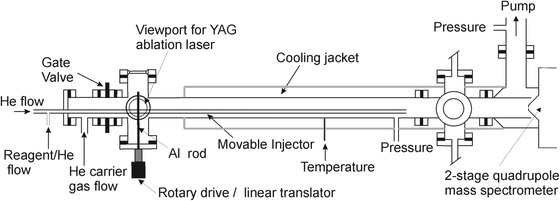Fig. 1 Schematic diagram of the fast flow tube with a laser ablation ion source, coupled to a differentially pumped quadrupole mass spectrometer.

Al+ ions were produced via laser ablation of a solid Al rod using a 532 nm Nd:YAG laser (repetition rate = 10 Hz, pulse energy ∼25 mJ), loosely focused onto the target using a quartz lens (focal length = 150 mm). The ablation target was mounted on a rotary feedthrough powered by a DC motor, and extended into the centre of the cylindrical axis of the tube (Fig. 1). The target was rotated at 2–4 Hz so that a fresh Al surface was presented to each laser pulse in order to maintain a uniform Al+ signal. The Al+ ion pulses were entrained in a flow of He which entered upstream of the ablation target. An overall gas flow rate of typically 4200 sccm was used at pressures of 1–4 Torr, controlled by a throttle valve situated on the exhaust. The resulting flow velocities ranged from 55–14 m s−1 and hence the Reynolds number was always less than 80, ensuring laminar flow within the tube.

The reactants were injected into the flow tube through a sliding glass injector positioned along the floor of the tube (Fig. 1). To generate O3, O2 was passed through a high voltage corona in a commercial ozoniser (Fischer Technology Ozone Generator 500 Series), producing a 5–8% mixture of O3 in O2. The O3 concentration was monitored in a 30 cm pathlength optical cell downstream of the ozonizer, by optical absorption of the 253.7 nm emission line from a Hg lamp.

Al+ and product molecular ions were detected using a differentially pumped 2-stage quadrupole mass spectrometer run in positive ion mode (Hiden Analytical, HPR60). The skimmer cone between the flow tube and the first stage of the mass spectrometer had a 0.4 mm orifice biased at −17 V, and the skimmer cone between the first and second stage of the mass spectrometer had a 1.8 mm orifice biased at −86 V. Time-resolved ion pulses were captured with a multichannel scaler synchronized to the Q switch of the YAG laser using a digital delay generator (Quantum model 9518). Al+ pulses from typically 500–1000 laser shots were then signal-averaged.

### Materials

Carrier gas He (99.995%, BOC gases) was purified by passing through molecular sieve (4 Å, 1–2 mm, Alfa Aesar) held at 77 K. N2 (99.995%, BOC gases), CO2 (99.995%, BOC gases), and O2 (99.999%, BOC gases) were used without purification. H2O and CO2 vapour were purified by three freeze–pump–thaw cycles before making up mixtures in He on an all-glass vacuum line.

## 3. Experimental results

Laser ablation/ionization of the Al rod most likely produces some Al+ ions in excited states. If these were sufficiently long-lived metastable states, they might affect the kinetic measurements (and would be recorded as m/z = 27 by the mass spectrometer). The metastable state that would potentially be a problem is Al+(33P), which is 4.64 eV above the Al+(31S) ground state. However, its radiative lifetime is 304 μs,21 which is much shorter than the flow time from the ablation source to the point where reactants were injected (typically 5–40 ms, depending on the reaction distance and flow rate). Thus, essentially all of these metastable ions would have radiated (or been quenched) to Al+(1S) before the introduction of the reactants.

The kinetics measurements were generally made by adjusting the injector length in the tube, so that reactants were injected from between 110 and 430 mm upstream of the skimmer cone of the mass spectrometer. The loss of Al+ by reactions (R1)–(R5) can be described by a pseudo first-order decay coefficient, k′, since the concentrations of the reactants, and the bath gas in the case of reactions (R2)–(R5), were in large excess of the Al+ concentration. Diffusional loss of Al+ to the tube walls, kdiff,Al+, is also first-order. Thus, in the case of reaction (R1) the total removal of Al+ is given by:

 ktotal′ = (kdiff,Al+ + k1[O3] + k3[O2][M]) (I)
and for reactions (R2)–(R5):
 ktotal′ = (kdiff,Al+ + kX[X]) (II)
where kdiff,Al+ is the first-order loss of the ion on the walls and kX is the pressure-dependent rate coefficient for the recombination of Al+ with X = N2, O2, CO2 or H2O. Eqn (I) includes the recombination of Al+ with O2 since this species was always present in the O3 flow, although in this case k3 is extremely slow (see below) and so in practice could be ignored. In the absence of reactants, the Al+ concentration at the skimmer cone, [Al+]t0, is given by:
 ln[Al+]t0 = ln[Al+]t=0 − t·kdiff,Al+ (III)
where [Al+]t=0 is the Al+ concentration at the injection point of X, a flow time t upstream of the skimmer cone. When reactant X is added, the Al+ density at the skimmer cone is given by:
 ln[Al+]tX = ln[Al+]t=0 − t(kdiff,Al+ + kX[X]) (IV)
Subtracting eqn (III) from (IV) produces an expression for k′ which describes reactive loss of Al+ only:
 (V)
The advantage of using eqn (V) is that the wall loss rate of Al+ is not required to obtain k′. Note that the flow times t referred to below are corrected for the parabolic velocity profile in the flow tube,22 which arises under laminar flow conditions when a reactant is removed rapidly at the walls. The velocity along the axis of the tube, which is where the ions are sampled, is 1.6 times the plug flow velocity – confirmed in the present experiment by measuring the arrival time of the pulse at the skimmer cone. It should be noted that the voltage on the first skimmer cone was set to maximize the ion signal, and at −17 V is high enough to potentially cause significant dissociation of weakly-bound cluster ions entering the mass spectrometer. If that were the case, a residual Al+ signal would be observed at long reaction times, and a plot of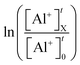versus t would not remain linear. However, modelling the kinetic measurements discussed below shows that less than 0.2% of clusters such as Al+·CO2 dissociated while passing through the skimmer cone.

### 3.1 Diffusion of Al+

Before making kinetic measurements of Al+ reactions, diffusional loss of Al+ to the flow tube walls was examined. Fig. 2 shows a sequence of Al+ pulses arriving at the mass spectrometer under conditions of constant pressure and varied flow times (note that the time shown in Fig. 2 is from the ablation source to the skimmer cone, which is longer than the reaction times where reactants are injected downstream of the source). The flight time is dependent on the flow velocity. At longer flight times, the pulse widths increase as a result of axial diffusion, and the pulse height and integrated area decrease due to radial diffusion and loss on the walls. A log-plot of the integrated pulse area against flight time yields a linear plot (Fig. 2) with a slope equal to the first-order loss on the walls, kdiff,Al+. This can then be related to the diffusion coefficient of Al+ in He by the expression for diffusion out of a cylinder:23
 (VI)
where P is the flow tube pressure and r is the tube radius, and the equality would hold if the ion was removed with 100% efficiency on collision with the flow tube wall. Eqn (VI) yields DAl+–He ≥ 146 Torr cm2 s−1. In comparison, DAl+–He can be estimated to be 221 Torr cm2 s−1 at 298 K from the expression:24
 (VII)
where n is the concentration of He, kB the Boltzmann constant, α the polarizability of He (0.205 Å3), e the elemental charge and μ is the reduced mass of the Al+–He collision. The experimental lower limit is reasonably close to this estimate, which suggests efficient removal on the electrically earthed tube walls. The removal probability may be somewhat reduced through the walls becoming coated over time with a partially insulating metal oxide layer.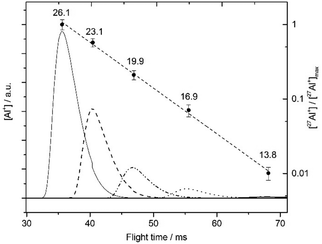Fig. 2 Al+ ion pulses (left-hand ordinate) recorded for five flow velocities (shown as numbers in m s−1 above each peak) at 3 Torr pressure of He. The points (with 1σ error bars determined from 3 repeated measurements) are the ratio of each pulse area to that of the pulse at 26 m s−1 (right-hand ordinate, log scale). The line is a linear regression through the points.

### 3.2 Al+ + O3

The data-points in Fig. 3 depicted with solid circles are an initial measurement of k1 yielding (1.6 ± 0.9) × 10−10 cm3 molecule−1 s−1. Note, however, that the linear regression line through these points does not pass through the origin as predicted by eqn (V). This is indicative of a reaction between AlO+ and O3 which recycles AlO+ to Al+ (reaction (R6a)), and retards the overall removal of Al+ at higher [O3]. This phenomenon has been reported recently for the analogous reaction Fe+ + O3.25 We therefore added H2O along with the O3 to remove AlO+ via reaction (R7), and hence inhibit recycling of AlO+ to Al+ via (R6a). Fig. 4 shows the effect on k′ of increasing [H2O] at three different fixed [O3]. k′ initially increases, but essentially reaches a plateau when more than ∼2 × 1012 molecule cm−3 of H2O is added. The measurement of k1 as a function of [O3] was now repeated at fixed [H2O] = 3.5 × 1012 cm−3, and the resulting k′ values (open triangles) are shown in Fig. 3. A regression through these points now passes through the origin, yielding k1 = (1.4 ± 0.1) × 10−9 cm3 molecule−1 s−1, where the uncertainty is the 1σ standard error of the regression slope combined with the uncertainty in the O3 concentration.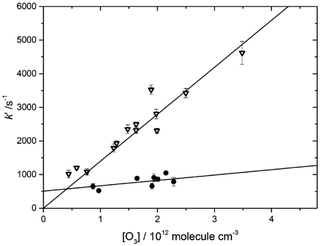Fig. 3 Plot of k′ versus [O3] for the study of reaction (R1) (O3). Data-points shown with open triangles are measured with a fixed [H2O] = 3.5 × 1012 molecule cm−3; data-points depicted with solid circles are measured in the absence of H2O.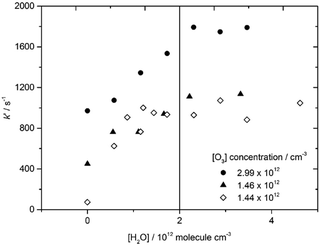Fig. 4 k′ versus [H2O] at three fixed [O3] (see figure legend). The vertical line indicates the point at which reaction (R7) dominates (R6a) so that k′ reaches a plateau and no longer increases with [H2O].

### 3.3 Al+ + CO2, N2, O2 and H2O

Fig. 5 shows plots ofversus t at three different He pressures for reaction (R4) (CO2). These plots are linear and pass through the origin, as expected from eqn (V). The slope of each plot yields the second-order recombination rate coefficient, krec, which is clearly increasing with pressure. Fig. 6 shows plots of krec versus [He] for (R4) (CO2) at two different temperatures. The slopes of these plots yield: k4(206 K) = (1.5 ± 0.2) × 10−29 cm6 molecule−2 s−1 and k4(293 K) = (5.0 ± 0.6) × 10−30 cm6 molecule−2 s−1.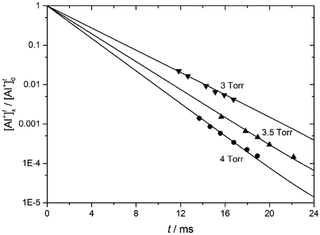Fig. 5 First-order decays of Al+ in the presence of CO2 ([CO2] = 6.0 × 1014 molecule cm−3) at 293 K, at three different pressures of He.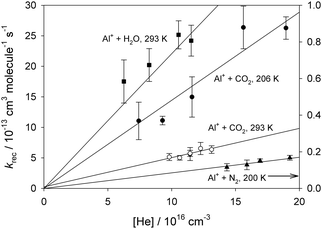Fig. 6 Second-order rate coefficients krec versus [He] for the recombination of Al+ with H2O, CO2 and N2 (note the right-hand ordinate for the N2 reaction).

The reaction Al+ + H2O was only measured at room temperature because of the constraint of H2O condensing on the flow tube walls at lower temperatures. As shown in Fig. 6, (R5) (H2O) has the largest rate coefficient of the four recombination reactions, with k5(293 K) = (2.4 ± 0.3) × 10−29 cm6 molecule−2 s−1. For Al+ + N2, the reaction is very slow and could only be observed at 200 K (note that the right-hand ordinate in Fig. 6 corresponds to (R2) (N2)), giving k2(200 K) = (8.4 ± 0.9) × 10−32 cm6 molecule−2 s−1. The uncertainties in these rate coefficients are the 1σ standard errors of the regression slopes combined with the uncertainty in the reactant concentrations. The reaction of Al+ + O2 was too slow to measure even at low temperatures, so an upper limit of k3(205 K) < 2.8 × 10−32 cm6 molecule−2 s−1 was obtained.

### 3.4 AlO+ + O3 and H2O

The removal of Al+ in the presence of O3 was studied as a function of t at 293 K. Four data-sets (with 6 to 10 points in each) of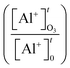versus t were obtained, as shown in Fig. 7. A model of the flow tube kinetics, which included k1, the wall loss of Al+, and the two unknowns k6 and the branching ratio f6a (where f6a = k6a/k6 i.e. the fraction of the total removal rate that is due to recycling to Al+), was then used to fit each data set by minimizing the χ2 residual between the modelled and experimental points. This yielded weighted means of k6(293 K) = (1.3 ± 0.6) × 10−9 cm3 molecule−1 s−1 and f6a = (63 ± 9)% (1σ uncertainty). The fitting procedure is much more sensitive to f6a than k6, because it is the branching ratio that dictates the amount of Al+ present rather than the absolute rate of reaction of AlO+ with O3. This is indicated by the relative uncertainties of the two parameters.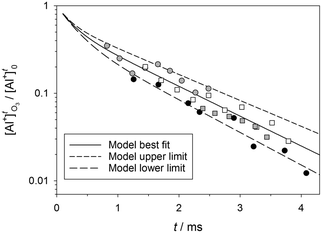Fig. 7 log plot ofas a function of t, with [O3] = 1.2 × 1012 cm−3 at 1.0 Torr and 293 K. The model fit is the solid black line, with upper and lower limits indicated by the dashed lines. Four experimental data sets are shown as discrete symbols.

The reaction between AlO+ and H2O was studied by monitoring the AlO+ ion with the mass spectrometer, as a function of [H2O] at fixed [O3]. Plots of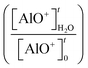versus t were then fitted with the kinetic model using the measured k1, k6a and k6b. Fitting four data sets with 5 to 10 data-points each resulted in a value of k7(293 K) = (9 ± 4) × 10−10 cm3 molecule−1 s−1. Note that we assume here that if reaction (R1)(O3) does indeed produce excited AlO+(3Π), because this state is only a few kJ mol−1 above AlO+(X1Σ) (see Section 1) it will be efficiently quenched at the pressures of He in the flow tube, before going on to react with O3 or H2O.

## 4. Discussion

A series of electronic structure calculations were first performed in order to interpret the experimental results. The geometries of AlO+ and the Al+ cluster ions were first optimized at the B3LYP/6-311+g(2d,p) level of theory within the Gaussian 16 suite of programs.26 The geometries are illustrated in Fig. 8, and the Cartesian coordinates, rotational constants and vibrational frequencies are listed in Table 1. The bond energies of the molecules (i.e. D°(Al+–X)) were then calculated using the more accurate CBS-QB3 method.13 Note that the cluster bond energies decrease in the order H2O > CO2 > N2 > O2, and inspection of Fig. 8 shows that the Al+–ligand bond length varies inversely with the bond energy, as expected.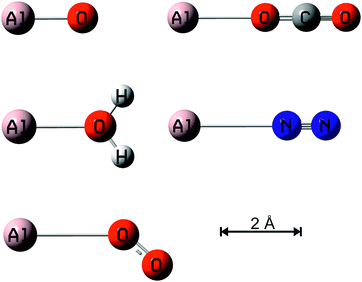Fig. 8 Geometries of AlO+, Al+·CO2, Al+·H2O, Al+·N2 and Al+·O2 ions (all singlet spin multiplicity) calculated at the B3LYP/6-311+g(2d,p) level of theory.26
Table 1 Molecular properties of the AlO+, Al+·CO2, Al+·H2O, Al+·N2 and Al+·O2 ions (illustrated in Fig. 8), and Al+–ligand bond energies
Molecule Geometry (Cartesian co-ordinates in Å)a Rotational constantsa (GHz) Vibrational frequenciesa (cm−1) D°(0 K)b (kJ mol−1)
a Calculated at the B3LYP/6-311+g(2d,p) level of theory.26b Calculated at the CBS-QB3 level of theory.13c Dissociation to Al+ + O(1D).d Dissociation to Al+ + O(3P).
AlO+ (X1Σ) Al, 0.0, 0.0, 0.0 19.5551 1001 326.9c
O, 0.0, 0.0, 1.604
AlO+ (a3Π) Al, 0.0, 0.0, 0.0 16.4096 726 115.4d
O, 0.0, 0.0, 1.751
Al+·CO2 Al, 0.0, 0.0, −0.175 2.0074 41 (×2), 136, 648 (×2), 1353, 2415 44.6
O, 0.0, 0.0, 2.191
C, 0.0, 0.0, 3.371
O, 0.0, 0.0, 4.513
Al+·H2O Al, −0.005, −0.028, −0.219 408.4034 292, 342, 496, 1647, 3688, 3775 111.4
O, 0.014, 0.077, 1.886 9.8024
H, 0.790, −0.030, 2.462 9.5726
H, −0.753, 0.242, 2.462
Al+·N2 Al, 0.0, 0.0, 1.783 2.9858 105 (×2), 108, 2439 18.9
N, 0.0, 0.0, −1.093
N, 0.0, 0.0, −2.184
Al+·O2 Al, −0.295, 0.0, −0.441 140.46524 72, 122, 1618 12.1
O, 0.258, 0.0, 2.256 3.14751
O, 1.229, 0.0, 2.974 3.07853

There is very good agreement for the bond lengths and vibrational frequencies of AlO+(X1Σ) and AlO+(a3Π) with two recent multireference configuration interaction theory studies.10,17 The difference in energy between these AlO+ states is 10.1 kJ mol−1 at the CBS-QB3 level, compared with 3.5–8.0 kJ mol−1 in these earlier studies i.e. well within the expected uncertainty. The AlO+(X1Σ) bond dissociation energy of 125 kJ mol−1 at the CBS-QB3 level lies between the previous theoretical estimates of 84 kJ mol117 and 140 kJ mol−1,10 and is somewhat lower than the experimental values of 132 and 145 kJ mol−1 obtained from beam studies of Al+ + NO2 and O2, respectively.16

### 4.1 Al+ + O3, AlO+ + O3 and H2O

Reaction (R1) (O3) is around 40% faster than the Langevin capture rate of 1.0 × 10−9 cm3 molecule−1 s−1, which indicates that the modest dipole moment of O3 (0.53 D11) enhances its capture by Al+. Using the statistical adiabatic channel model (SACM) of Troe27 with a rotational constant for O3 of 0.428 cm−1, estimated as the geometric mean of the rotation constants for rotation orthogonal to the C2v axis of the molecule along which the dipole lies, yields k1(293 K) = 1.39 × 10−9 cm3 molecule−1 s−1, in excellent agreement with the measured value. The temperature dependence of the reaction is then predicted to be k1(100–300 K) = 1.48 × 10−9 (T/200)−0.164 cm3 molecule−1 s−1. Note that the large value of k1 confirms that the AlO+ bond energy must be greater than D°(O–O2) (= 100 kJ mol−111), which is consistent with Sghaier et al.10 and the present study, and not with the calculation of Yan et al.17

For the reaction of AlO+ with O3, both channels are substantially exothermic (Section 1), so it is perhaps not surprising that the branching to Al+ and OAlO+ is evenly split, with f6a = 63%. Application of the SACM estimates k6(100–300 K) = 1.20 × 10−9 (T/293)−0.175 cm3 molecule−1 s−1, which agrees well with the measured value within the experimental uncertainty. For (R7), the larger dipole moment of H2O increases the SACM estimate to k7(100–300 K) = 2.30 × 10−9 (T/293)−0.309 cm3 molecule−1 s−1, which is about double the measured value.

### 4.2 Al+ + N2, O2, CO2 and H2O

In order to extrapolate the rate coefficients for these cluster reactions to temperatures and pressures which were not accessible experimentally, we employed Rice–Ramsperger–Kassel–Markus (RRKM) theory using a solution of the Master Equation (ME) based on the inverse Laplace transform method.28 We have applied this formalism previously to recombination reactions of metallic species18,20,29 so only a brief description is given here. These reactions proceed via the formation of an excited adduct, which can either dissociate or be stabilized by collision with the third body. The internal energy of this adduct was divided into a contiguous set of grains (width 5–30 cm−1, depending on the well depth of the adduct), each containing a bundle of rovibrational states. Each grain was then assigned a set of microcanonical rate coefficients for dissociation, which were determined using inverse Laplace transformation to link them directly to krec,∞, the high pressure limiting recombination coefficient which was estimated here using Langevin capture theory (including a correction for the permanent dipole of H2O in the case of (R5) (H2O)). Using the vibrational frequencies and rotational constants listed in Table 1, the density of states of each adduct was calculated with the Beyer–Swinehart algorithm for the vibrational modes (without making a correction for anharmonicity), and a classical densities of states treatment for the rotational modes.30

The probability of collisional transfer between grains was estimated using the exponential down model, where the average energy for downward transitions is designated 〈ΔEdown.30 The probabilities for upward transitions were calculated by detailed balance. The ME describes the evolution with time of the adduct grain populations. The ME was expressed in matrix form and then solved to yield the recombination rate constant at a specified pressure and temperature. When fitting to the experimental data, three adjustable parameters were allowed: the average energy for downward transitions, 〈ΔEdown; α, which defines the Tα dependence of 〈ΔEdown; and a barrier of height V0 for cases where vibrational modes with low frequencies were more correctly treated as hindered rotors.30 Table 2 summarises the results. The fitted values of 〈ΔEdown lie between 100 and 160 cm−1, which is the expected range for He.30 For (R4) (CO2), the two low-frequency degenerate vibrational modes of Al+–CO2 (41 cm−1) were treated as a 2-dimensional rotor with V0 = 3 kJ mol−1. The fitted value of α is −0.2, which is close to 0 as expected.30 For (R5) (H2O), the out-of-plane and in-plane rocking modes of the Al+–H2O cluster (342 and 496 cm−1) were treated as a 2-dimensional rotor with V0 = 2 kJ mol−1.

Table 2 Fitted RRKM parameters and low-pressure limiting rate coefficients for the addition of a single ligand to an Al+ ion
Reaction 〈ΔEdown (cm−1) α log10(krec,0/cm6 molecule−2 s−1) T = 100–600 K
a Assumed T dependence.
Al+ + N2 112 0.0a −27.9739 + 0.05036log10(T) − 0.60987(log10(T))2
Al+ + CO2 125 −0.2 −33.6387 + 7.0522log10(T) − 2.1467(log10(T))2
Al+ + H2O 155 0.0a −24.7835 + 0.018833log10(T) − 0.6436(log10(T))2

The resulting fits of the low pressure limiting rate coefficient, krec,0, through the experimental data points and then extrapolated between 100 and 600 K, are illustrated in Fig. 9. Reaction (R5) (H2O) is almost 1000 times faster than (R2) (N2) (at the same temperature). This reflects the much deeper well and the increased number of atoms in Al+–H2O compared with the Al+–N2 cluster: both factors increase the density of ro-vibrational states of the adduct. Note that for all three reactions krec,0 does not follow a simple Tn dependence, and so a second-order dependence on log10T was fitted in each case. The resulting expressions are listed in the final column of Table 2 (the large number of significant figures in the fitted polynomial parameters are provided for numerical accuracy). For (R4) (CO2), the fitted value of α lies between −0.3 and +0.1. Since (R2) (N2) and (R5) (H2O) were only measured at a single temperature, α was set to 0.0; this parameter is expected to lie between −0.5 and +0.5.30 The faint lines in Fig. 9 show the sensitivity of the RRKM fit for each reaction when α is varied between these respective limits. At a temperature of 180 K (typical of the terrestrial mesosphere2), the overall uncertainties in the rate coefficients combining the experimental error and RRKM extrapolation is then 12% for (R2) (N2), 13% for (R4) (CO2), and 27% for (R5) (H2O). Note that these low-pressure limiting rate coefficients are appropriate for the meteoric ablation region in a planetary atmosphere where the pressure is less than 10−5 bar.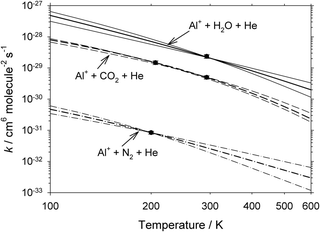Fig. 9 RRKM fits (thick lines) through the experimental data points (solid circles) for the recombination reactions of Al+ with N2, CO2 and H2O. The faint lines indicate the sensitivity of each fit to the likely range of α, the temperature-dependence of 〈ΔE〉down.

We now consider (R2) (N2) in more detail. Since D0(Al+–N2) is only 19 kJ mol−1 (Table 1), the RRKM calculations indicate that this cluster ion dissociates with an e-folding lifetime of 82 μs at 200 K and 4 Torr i.e. the reaction would not have been observed in the flow tube, unless a ligand such as CO2 or H2O switched with the N2 in order to stabilize the Al+–X cluster ion. We investigated this using the Master Equation Solver for Multi-Energy well Reactions (MESMER) program,31,32 which has the facility to include bimolecular removal of the Al+–N2 adduct to a stable sink. The switching rate coefficients for the reactions

 Al+·N2 + CO2 → Al+·CO2 + N2 ΔH° = −25 kJ mol−1 (VIII)
 Al+·N2 + H2O → Al+·H2O + N2 ΔH° = −92 kJ mol−1 (IX)
were set to their respective Langevin capture rates: k8(200 K) = 8.1 × 10−10 cm3 molecule−1 s−1, and k9(200 K) = 2.9 × 10−9 cm3 molecule−1 s−1, and the other parameters as above. This exercise reveals that Al+ ions would be removed at the observed rate if recombination with N2 was followed by switching, so long as the H2O mixing ratio in the N2 was above 2 ppm, or CO2 above 7 ppm (likely in 99.995% pure N2). In terms of atmospheric chemistry, in the terrestrial upper mesosphere the mixing ratio of CO2 is 390 ppm,33 so that (R2) (N2) needs to be taken into account as a potential route for neutralizing Al+.

Lastly, we note that for Al+ + O2, Leuchtner et al.34 found an upper limit of k3(300 K) ≤ 1.3 × 10−32 cm6 molecule−2 s−1 in a selected ion flow tube at a pressure of 0.25 Torr, which is consistent with the upper limit of 2.8 × 10−32 cm6 molecule−2 s−1 in the present study. For Al+ + CO2, Clemmer et al.16 reported k4(300 K) ≤ 2.0 × 10−27 cm6 molecule−2 s−1 in a guided ion beam instrument with a maximum pressure of 0.3 mTorr. This is consistent with our actual measurement of k4(293 K) = (5.0 ± 0.6) × 10−30 cm6 molecule−2 s−1.

### 4.3 Atmospheric implications

In order to use the cluster reaction rate coefficients for modelling in a planetary atmosphere, they need to be adjusted to account for the relative efficiencies of the major atmospheric species compared with the He used in the kinetic measurements. For N2 and O2 acting as a third body in an ion–molecule recombination reaction, the rate coefficients k2, k4 and k5 should typically be increased by a factor of 3,2 and for CO2 by a factor of 8.12 Fig. 10 illustrates vertical profiles for the removal of Al+ ions in the atmospheres of Earth and Mars. For Earth, the vertical profiles of T, pressure and the mixing ratios of O3, N2, CO2 and H2O are taken from the Whole Atmosphere Community Climate Model (WACCM4).35,36 They are monthly zonal averages at 40°N in April, at local midnight. Fig. 10 (top panel) shows that reaction (R1) (O3) dominates between 80 and 140 km. Reaction (R2) (N2) is actually more important than (R4) (CO2), because of the ability for the Al+·N2 ion to ligand-switch with CO2 (or H2O) before dissociating, though of course this is just an indirect route to forming Al+·CO2. It should be noted that similar ligand-switching reactions occur with the N2 clusters of NO+ and O2+ which are the major ions in the upper D region of the terrestrial ionosphere,37 as well as with Fe+·N218 and Mg+·N2.20 During daytime the O3 concentration decreases by around 1 order of magnitude due to photolysis,2 but (R1) (O3) will still dominate. (R5) (H2O) is least important because of the low mixing ratio of H2O, which is less than a few ppm above 80 km2.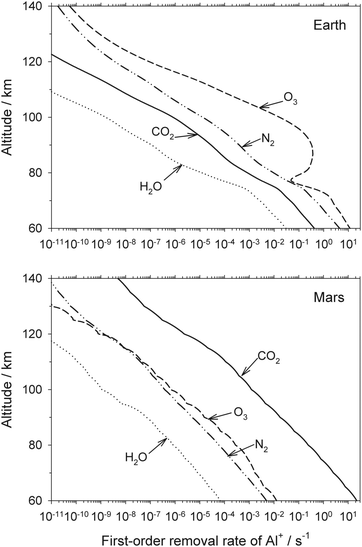Fig. 10 Removal rates of Al+ ions in planetary atmospheres: Earth, 40°N, local midnight, April (top panel); Mars, local noon, latitude = 0°, solar longitude Ls = 85° (bottom panel).

For Mars, the vertical profiles of the relevant species and T are taken from the Mars Climate Database v.5.2 (http://www-mars.lmd.jussieu.fr/mcd_python/),38 for the conditions of latitude = 0°, local noon and solar longitude Ls = 85° (northern hemisphere summer). Because the Martian atmosphere is ∼95% CO2, and the O3 concentration is much lower than in the terrestrial atmosphere (e.g. by a factor of 0.002 at 80 km), (R4) (CO2) dominates by about 3 orders of magnitude (Fig. 10, bottom panel).

On Earth, the metallic ion layers such as Fe+39 and Mg+40 peak around 95 km, where Fig. 10 (top panel) shows that the e-folding lifetime of Al+ is only ∼10 s. On Mars, recent measurements by the MAVEN spacecraft show that the Mg+ layer peaks around 90 km,41 where the e-folding lifetime of Al+ ions will be around 1 minute. Al+ would thus rapidly disappear on either planet. However, the reaction AlO+ + O → Al+ + O is likely to be fast (cf. the analogous reactions of MgO+,12 CaO+19 and FeO+42), and so AlO+ is much more likely to recycle to Al+ than to undergo dissociative recombination with an electron.43 CO may also play an analogous role to O in reducing AlO+ back to Al+.42 On Mars, AlO+ would likely be produced from the CO2 cluster ion by the reaction

 Al+·CO2 + O → AlO+ + CO2 ΔH° = −81 kJ mol−1 (X)

## 5. Conclusions

The kinetics of the reactions of Al+ with O3, N2, CO2 and H2O have been measured for the first time, and an upper limit obtained to recombination with O2. The Al+ ion is a closed-shell species and so relatively unreactive, forming comparatively weak bonds with CO2, N2 and particularly O2. Thus, while the recombination reaction with CO2 can be observed at room temperature, the N2 reaction can only be observed at lower temperatures and in the presence of a switching ligand like CO2 or H2O. In contrast, the spin-conserving reaction with O3 to form AlO+ in the low-lying a3Π triplet state is exothermic and fast, proceeding at close to the ion–molecule capture rate which is enhanced by the small dipole moment of O3. This reaction dominates removal of Al+ in the terrestrial atmosphere because of the relatively high concentration of O3 in the MLT, whereas on Mars recombination with CO2 is about 103 times faster.

## Conflicts of interest

There are no conflicts to declare.

## Acknowledgements

This work was supported by Natural Environment Research Council grant NE/P001815/1. S. M. D. was supported by a studentship from the NERC SPHERES Doctoral Training Program. We thank Wuhu Feng (University of Leeds and National Centre for Atmospheric Science) for providing the WACCM data used in Section 4.3. The data used in the paper is archived at the Leeds University PetaByte Environmental Tape Archive and Library (PETAL; http://www.see.leeds.ac.uk/business-and-consultation/facilities/petabyte-environmental-tapearchive-and-library-petal/), and is available from J. M. C. P.

## References

1. J. D. Carrillo-Sánchez, D. Nesvorný, P. Pokorný, D. Janches and J. M. C. Plane, Geophys. Res. Lett., 2016, 43, 11,979–911,986 CrossRef PubMed.
2. J. M. C. Plane, W. Feng and E. C. M. Dawkins, Chem. Rev., 2015, 115, 4497–4541 CrossRef CAS PubMed.
3. E. Jarosewich, Meteoritics, 1990, 25, 323–337 CrossRef CAS.
4. R. Hutchison, Meteorites: A Petrologic, Chemical and Isotopic Synthesis, Cambridge University Press, 2004 Search PubMed.
5. E. Kopp, J. Geophys. Res.: Space Phys., 1997, 102, 9667–9674 CrossRef CAS.
6. M. Benna, P. R. Mahaffy, J. M. Grebowsky, J. M. C. Plane, R. V. Yelle and B. M. Jakosky, Geophys. Res. Lett., 2015, 42, 4670–4675 CrossRef CAS.
7. M. DeLuca, T. Munsat, E. Thomas and Z. Sternovsky, Planet. Space Sci., 2018, 156, 111–116 CrossRef CAS.
8. J. C. Gómez Martín, S. M. Daly, J. S. A. Brooke and J. M. C. Plane, Chem. Phys. Lett., 2017, 675, 56–62 CrossRef.
9. M. E. Weber, J. L. Elkind and P. B. Armentrout, J. Chem. Phys., 1986, 84, 1521–1529 CrossRef CAS.
10. O. Sghaier, R. Linguerri, M. M. Al Mogren, J. S. Francisco and M. Hochlaf, Astrophys. J., 2016, 826, 163 CrossRef.
11. D. R. Lide, Handbook of Physics and Chemistry, CRC Press, Boca Raton, FL, 2006 Search PubMed.
12. C. L. Whalley and J. M. C. Plane, Faraday Discuss., 2010, 147, 349–368 RSC.
13. J. A. Montgomery, M. J. Frisch, J. W. Ochterski and G. A. Petersson, J. Chem. Phys., 2000, 112, 6532–6542 CrossRef CAS.
14. J. A. Rutherford, R. H. Neynaber and D. A. Vroom, Measurements of selected charge transfer processes at low energies: final report for period November 1977-November 1978 (contract No. DNA 001-77-C-0202), Defense Nuclear Agency Report, 1978 Search PubMed.
15. V. G. Anicich, J. Phys. Chem. Ref. Data, 1993, 22, 1469–1569 CrossRef CAS.
16. D. E. Clemmer, M. E. Weber and P. B. Armentrout, J. Phys. Chem., 1992, 96, 10888–10893 CrossRef CAS.
17. P. Y. Yan, X. Yuan, S. Yin, X. T. Liu, H. F. Xu and B. Yan, Comput. Theor. Chem., 2017, 1117, 258–265 CrossRef CAS.
18. T. Vondrak, K. R. I. Woodcock and J. M. C. Plane, Phys. Chem. Chem. Phys., 2006, 8, 503–512 RSC.
19. S. Broadley, T. Vondrak, T. G. Wright and J. M. C. Plane, Phys. Chem. Chem. Phys., 2008, 10, 5287–5298 RSC.
20. C. L. Whalley, J. C. Gomez Martin, T. G. Wright and J. M. C. Plane, Phys. Chem. Chem. Phys., 2011, 13, 6352–6364 RSC.
21. A. Kramida, Y. Ralchenko, J. Reader and the NIST ASD Team, NIST Atomic Spectra Database (ver.5.2), National Institute of Standards and Technology, Gaithersburg, MD, 2018, http://physics.nist.gov/asd.
22. R. L. Brown, J. Res. Natl. Bur. Stand., 1978, 83, 1–8 CrossRef CAS.
23. J. Crank, The Mathematics of Diffusion, Oxford Science Publications, Oxford, 1986 Search PubMed.
24. M. H. Rees, Physics and Chemistry of the Upper Atmosphere, Cambridge University Press, Cambridge, 1989 Search PubMed.
25. J. J. Melko, S. G. Ard, T. Lê, G. S. Miller, O. Martinez, N. S. Shuman and A. A. Viggiano, J. Phys. Chem. A, 2017, 121, 24–30 CrossRef CAS PubMed.
26. M. J. Frisch, G. W. Trucks, H. B. Schlegel, G. E. Scuseria, M. A. Robb, J. R. Cheeseman, G. Scalmani, V. Barone, G. A. Petersson, H. Nakatsuji, X. Li, M. Caricato, A. V. Marenich, J. Bloino, B. G. Janesko, R. Gomperts, B. Mennucci, H. P. Hratchian, J. V. Ortiz, A. F. Izmaylov, J. L. Sonnenberg, D. Williams-Young, F. Ding, F. Lipparini, F. Egidi, J. Goings, B. Peng, A. Petrone, T. Henderson, D. Ranasinghe, V. G. Zakrzewski, N. R. J. Gao, G. Zheng, W. Liang, M. Hada, M. Ehara, K. Toyota, R. Fukuda, J. Hasegawa, M. Ishida, T. Nakajima, Y. Honda, O. Kitao, H. Nakai, T. Vreven, K. Throssell, J. J. A. Montgomery, J. E. Peralta, F. Ogliaro, M. J. Bearpark, J. J. Heyd, E. N. Brothers, K. N. Kudin, V. N. Staroverov, T. A. Keith, R. Kobayashi, J. Normand, K. Raghavachari, A. P. Rendell, J. C. Burant, S. S. Iyengar, J. Tomasi, M. Cossi, J. M. Millam, M. Klene, C. Adamo, R. Cammi, J. W. Ochterski, R. L. Martin, K. Morokuma, O. Farkas, J. B. Foresman and D. J. Fox, Gaussian, Inc., Wallingford, CT, USA, 2016.
27. J. Troe, Chem. Phys. Lett., 1985, 122, 425–430 CrossRef CAS.
28. R. De Avillez Pereira, D. L. Baulch, M. J. Pilling, S. H. Robertson and G. Zeng, J. Phys. Chem., 1997, 101, 9681 CrossRef.
29. S. L. Broadley, T. Vondrak and J. M. C. Plane, Phys. Chem. Chem. Phys., 2007, 9, 4357–4369 RSC.
30. R. G. Gilbert and S. C. Smith, Theory of Unimolecular and Recombination Reactions, Blackwell, Oxford, 1990 Search PubMed.
31. D. R. Glowacki, C.-H. Liang, C. Morley, M. J. Pilling and S. H. Robertson, J. Phys. Chem. A, 2012, 116, 9545–9560 CrossRef CAS PubMed.
32. S. H. Robertson, D. R. Glowacki, C.-H. Liang, C. Morley, R. Shannon, M. Blitz and M. J. Pilling, MESMER (Master Equation Solver for Multi-Energy Well Reactions), http://sourceforge.net/projects/mesmer.
33. M. López-Puertas, B. Funke, A. A. Jurado-Navarro, M. Garcia-Comas, A. Gardini, C. D. Boone, L. Rezac and R. R. Garcia, J. Geophys. Res.: Atmos., 2017, 122, 8345–8366 Search PubMed.
34. R. E. Leuchtner, A. C. Harms and A. W. Castleman, J. Chem. Phys., 1991, 94, 1093–1101 CrossRef CAS.
35. D. R. Marsh, M. J. Mills, D. E. Kinnison, J.-F. Lamarque, N. Calvo and L. M. Polvani, J. Clim., 2013, 26, 7372–7391 CrossRef.
36. R. R. Garcia, D. Marsh, D. E. Kinnison, B. Boville and F. Sassi, J. Geophys. Res.: Atmos., 2007, 112, D09301 Search PubMed.
37. N. S. Shuman, D. E. Hunton and A. A. Viggiano, Chem. Rev., 2015, 115, 4542–4570 CrossRef CAS PubMed.
38. F. Forget, F. Hourdin, R. Fournier, C. Hourdin, O. Talagrand, M. Collins, S. R. Lewis, P. L. Read and J.-P. Huot, J. Geophys. Res., 1999, 104, 24155–24176 CrossRef CAS.
39. W. Feng, D. R. Marsh, M. P. Chipperfield, D. Janches, J. Höffner, F. Yi and J. M. C. Plane, J. Geophys. Res., 2013, 118, 9456–9474 CAS.
40. M. P. Langowski, C. von Savigny, J. P. Burrows, W. Feng, J. M. C. Plane, D. R. Marsh, D. Janches, M. Sinnhuber, A. C. Aikin and P. Liebing, Atmos. Chem. Phys., 2015, 15, 273–295 CrossRef.
41. M. M. J. Crismani, N. M. Schneider, J. M. C. Plane, J. S. Evans, S. K. Jain, M. S. Chaffin, J. D. Carrillo-Sanchez, J. I. Deighan, R. V. Yelle, A. I. F. Stewart, W. McClintock, J. Clarke, G. M. Holsclaw, A. Stiepen, F. Montmessin and B. M. Jakosky, Nat. Geo., 2017, 10, 401–404 CrossRef CAS.
42. K. R. S. Woodcock, T. Vondrak, S. R. Meech and J. M. C. Plane, Phys. Chem. Chem. Phys., 2006, 8, 1812–1821 RSC.
43. D. L. Bones, J. M. C. Plane and W. Feng, J. Phys. Chem. A, 2016, 120, 1369–1376 CrossRef CAS PubMed.
 This journal is © the Owner Societies 2019